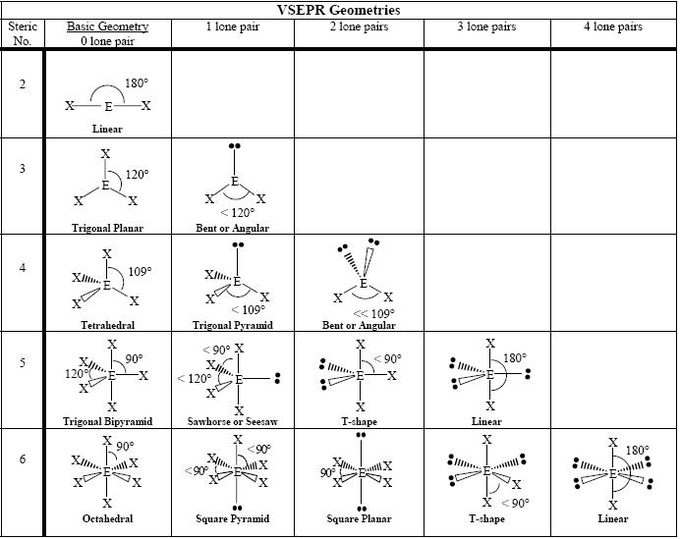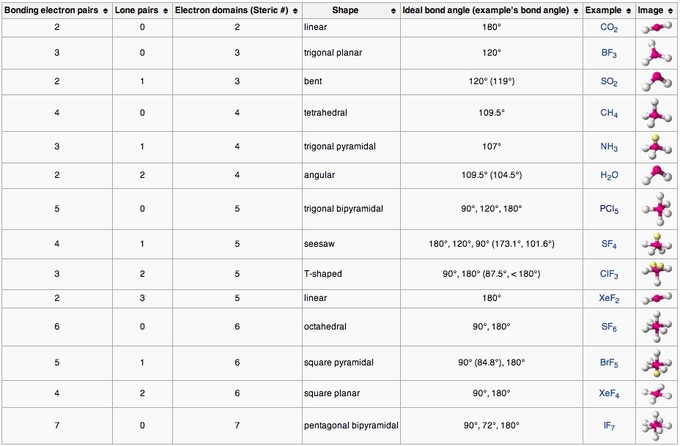## Table of Geometries

#### Learning Objective

• Apply the VSEPR model to determine the geometry of a molecule that contains no lone pairs of electrons on the central atom.

#### Key Points

• Fundamentally, the VSEPR model theorizes that regions of negative electric charge will repel each other, causing them (and the chemical bonds that they form) to stay as far apart as possible.
• Molecular geometries take into account the number of atoms and the number of lone pair electrons.
• The main geometries without lone pair electrons are: linear, trigonal, tetrahedral, trigonal bipyramidal, and octahedral.

#### Term

• VSEPR Theorya chemistry model used to predict the shape of individual molecules based on electron-pair electrostatic repulsion

## VSEPR Model

The valence shell electron pair repulsion (VSEPR) model focuses on the bonding and nonbonding electron pairs present in the outermost (valence) shell of an atom that connects with two or more other atoms.

Fundamentally, the VSEPR model theorizes that these regions of negative electric charge will repel each other, causing them (and the chemical bonds that they form) to stay as far apart as possible. Therefore, the two electron clouds contained in a simple triatomic molecule AX2 will extend out in opposite directions. An angular separation of 180° places the two bonding orbitals as far away from each other as possible; we therefore expect the two chemical bonds to extend in opposite directions, producing a linear molecule .Linear electron geometryThis ball-and-stick model represents a linear compound for formula AX2. The two X atoms (in white) are 180° away from one another.

If the central atom also contains one or more pairs of non-bonding electrons, these additional regions of negative charge will behave much like those associated with the bonded atoms. The orbitals containing the various bonding and non-bonding pairs in the valence shell will extend out from the central atom in directions that minimize their mutual repulsions. If the central atom possesses partially occupied d-orbitals, it may be able to accommodate five or six electron pairs, forming what is sometimes called an “expanded octet.”

## Molecular Geometries

Molecular geometries (linear, trigonal, tetrahedral, trigonal bipyramidal, and octahedral) are determined by the VSEPR theory. A table of geometries using the VSEPR theory can facilitate drawing and understanding molecules. The table of molecular geometries can be found in the first figure. The second figure serves as a visual aid for the table.VSEPR geometriesA visual guide to molecular geometries using the VSEPR Theory.VSEPR table of molecular geometriesThe bonded angles in the table are ideal angles from the simple VSEPR theory; the actual angle for the example given is in the following column.